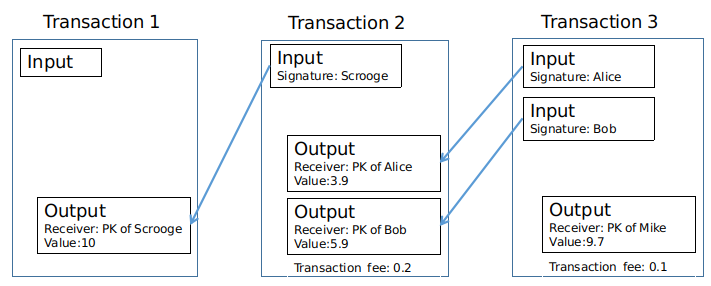# Programming Assignment: Scrooge Coin

## Bitcoin and Cryptocurrency Technologies-Week 1

Posted by 赵化冰 on Sunday, May 20, 2018

This series of articles are my notes of “Bitcoin and Cryptocurrency Technologies” online course.

Finally, I got to the most exciting part of week 1 lectures-the programming assignment!

I’m supposed to submit the assignment earlier because it was due a few weeks ago, however, I’d like to keep my pace relatively slow. I can’t invest my full time to this course because I have a job to do, so I only take this course in my spare time. I also would like to digest all the information in one lesson before moving on to the next. Some fundamental technologies such as hash function, hash pointer, blockchain, Merkel tree and digital signature have been well-explained in week 1 lectures. In order to better understand these technologies, I also did some searches and programming practices, which can be found in my previous posts.

It turns out that writing posts on my blog is a better way to learn, I have to fully understand the lessons before I can explain them in my posts.

## Scrooge Coin Transaction

Scrooge Coin programming assignment is a little bit tricky, the video of this lesson hasn’t explained some implementation details. To help you understand the transaction data structure used in Scrooge Coin, I draw this diagram:Every transaction has a set of inputs and a set of outputs. An input in a transaction must use a hash pointer to refer to its corresponding output in the previous transaction, and it must be signed with the private key of the owner because the owner needs to prove he/she agrees to spend his/her coins.

Every output is correlated to the public key of the receiver, which is his/her ScroogeCoin address.

In the first transaction, we assume that Scrooge has created 10 coins and assigned them to himself, we don’t doubt that because the system-Scroogecoin has a building rule which says that Scrooge has right to create coins.

In the second transaction, Scrooge transferred 3.9 coins to Alice and 5.9 coins to Bob. The sum of the two outputs is 0.2 less than the input because the transaction fee was 0.2 coin.

In the third transaction, there were two inputs and one output, Alice and Bob transferred 9.7 coins to mike, and the transaction fee was 0.1 coin.

## Unclaimed transaction outputs pool

Another trick we need to Note when doing the programming assignment is that an UTXOPool is introduced to track the unclaimed outputs (unspent coins), so we can know whether the corresponding output of an input of the transaction is available or not.

## TxHandler Java Code

``````import java.security.PublicKey;
import java.util.ArrayList;
import java.util.HashSet;
import java.util.List;
import java.util.Set;

public class TxHandler {
private UTXOPool utxoPool;

/**
* Creates a public ledger whose current UTXOPool (collection of unspent
* transaction outputs) is {@code utxoPool}. This should make a copy of utxoPool
* by using the UTXOPool(UTXOPool uPool) constructor.
*/
public TxHandler(UTXOPool utxoPool) {
this.utxoPool = new UTXOPool(utxoPool);
}

/**
* @return true if: (1) all outputs claimed by {@code tx} are in the current
*         UTXO pool, (2) the signatures on each input of {@code tx} are valid,
*         (3) no UTXO is claimed multiple times by {@code tx}, (4) all of
*         {@code tx}s output values are non-negative, and (5) the sum of
*         {@code tx}s input values is greater than or equal to the sum of its
*         output values; and false otherwise.
*/
public boolean isValidTx(Transaction tx) {
Set<UTXO> claimedUTXO = new HashSet<UTXO>();
double inputSum = 0;
double outputSum = 0;

List<Transaction.Input> inputs = tx.getInputs();
for (int i = 0; i < inputs.size(); i++) {
Transaction.Input input = inputs.get(i);

if (!isConsumedCoinAvailable(input)) {
return false;
}

if (!verifySignatureOfConsumeCoin(tx, i, input)) {
return false;
}

if (isCoinConsumedMultipleTimes(claimedUTXO, input)) {
return false;
}

UTXO utxo = new UTXO(input.prevTxHash, input.outputIndex);
Transaction.Output correspondingOutput = utxoPool.getTxOutput(utxo);
inputSum += correspondingOutput.value;

}

List<Transaction.Output> outputs = tx.getOutputs();
for (int i = 0; i < outputs.size(); i++) {
Transaction.Output output = outputs.get(i);
if (output.value <= 0) {
return false;
}

outputSum += output.value;
}

// Should the input value and output value be equal? Otherwise the ledger will
// become unbalanced.
// The difference between inputSum and outputSum is the transaction fee
if (outputSum > inputSum) {
return false;
}

return true;
}

private boolean isCoinConsumedMultipleTimes(Set<UTXO> claimedUTXO, Transaction.Input input) {
UTXO utxo = new UTXO(input.prevTxHash, input.outputIndex);
}

private boolean verifySignatureOfConsumeCoin(Transaction tx, int index, Transaction.Input input) {
UTXO utxo = new UTXO(input.prevTxHash, input.outputIndex);
Transaction.Output correspondingOutput = utxoPool.getTxOutput(utxo);
return Crypto.verifySignature(pk, tx.getRawDataToSign(index), input.signature);
}

private boolean isConsumedCoinAvailable(Transaction.Input input) {
UTXO utxo = new UTXO(input.prevTxHash, input.outputIndex);
return utxoPool.contains(utxo);
}

/**
* Handles each epoch by receiving an unordered array of proposed transactions,
* checking each transaction for correctness, returning a mutually valid array
* of accepted transactions, and updating the current UTXO pool as appropriate.
*/
public Transaction[] handleTxs(Transaction[] possibleTxs) {
List<Transaction> acceptedTx = new ArrayList<Transaction>();
for (int i = 0; i < possibleTxs.length; i++) {
Transaction tx = possibleTxs[i];
if (isValidTx(tx)) {

removeConsumedCoinsFromPool(tx);
}
}

Transaction[] result = new Transaction[acceptedTx.size()];
acceptedTx.toArray(result);
return result;
}

List<Transaction.Output> outputs = tx.getOutputs();
for (int j = 0; j < outputs.size(); j++) {
Transaction.Output output = outputs.get(j);
UTXO utxo = new UTXO(tx.getHash(), j);
}
}

private void removeConsumedCoinsFromPool(Transaction tx) {
List<Transaction.Input> inputs = tx.getInputs();
for (int j = 0; j < inputs.size(); j++) {
Transaction.Input input = inputs.get(j);
UTXO utxo = new UTXO(input.prevTxHash, input.outputIndex);
utxoPool.removeUTXO(utxo);
}
}

}
``````

## All the Example Codes on GitHub

I wrap the codes into a maven project, just run `mvn test` then the example codes will build and run all the test cases.

Scrooge Coin example in Java